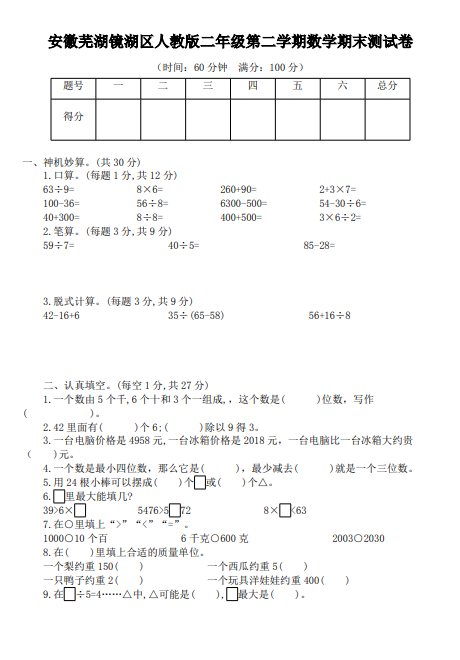ceomax主题已启用，当前站点还没有验证正版主题授权，暂不可使用 前往授权激活 获取正版授权
【PDF文档4页】安徽芜湖镜湖区人教版小学二年级第二学期数学期末测试卷含答案A4电子版资料_可直接打印_会员免费下载 - 悟思学_领悟_思考_学习

【PDF文档4页】安徽芜湖镜湖区人教版小学二年级第二学期数学期末测试卷含答案A4电子版资料_可直接打印_会员免费下载

215

（时间：60 分钟 满分：100 分）

1.口算。(每题 1 分,共 12 分)
63÷9= 8×6= 260+90= 2+3×7=
100-36= 56÷8= 6300-500= 54-30÷6=
40+300= 8÷8= 400+500= 3×6÷2=
2.笔算。(每题 3 分,共 9 分)
59÷7= 40÷5= 85-28=

【PDF文档4页】安徽芜湖镜湖区人教版小学二年级第二学期数学期末测试卷含答案A4电子版资料_可直接打印_会员免费下载相关资料203 5241 53341

• 0 +

访问总数

• 0 +

会员总数

• 0 +

文档总数

• 0 +

今日发布

• 0 +

本周发布

• 0 +

运行天数